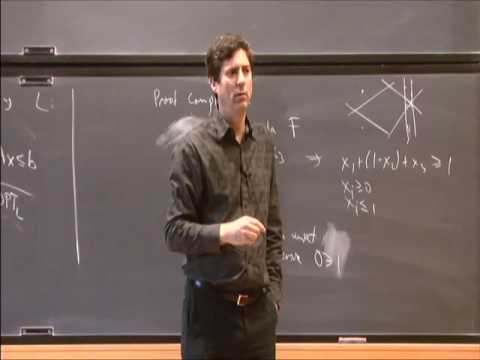## Hardness Escalation and the Rank of Polynomial Threshold Proofs

November 30, 2010
IAS
Presenters: Paul Beame

Watch Video

#### Abstract

A hardness escalation method applies a simple transformation that increases the complexity of a computational problem. Using multiparty communication complexity we present a generic hardness escalation method that converts any family of unsatisfiable CNF formulas that require large rank resolution proofs into CNF formulas whose refutation requires large rank for proofs using polynomial threshold functions of degree at most \$k\$ (known as \$Th(k)\$ proofs). Such systems include: Lovasz-Schrijver and Cutting Planes proof systems as well as their high degree analogues: \$LS(k)\$, \$LS+(k)\$, and \$CP(k)\$. Our results apply for all \$k\$ up to \$O(loglog n)\$ where \$n\$ is the number of variables in the CNF formulas. These yield strong rank lower bounds for polynomial threshold proofs for large classes of natural CNF formulas. We also show exponential lower bounds on the sizes of polynomial threshold proof trees for these formulas.Finally, we use our framework to present a general method for producing integrality gaps for sound low rank inference over linear inequalities, including optimal integrality gaps for \$MAX-2t-SAT\$.Joint work with Trinh Huynh and Toniann Pitassi.